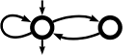Awali Another Weighted Automata library
abstract_ratexp.hh
Go to the documentation of this file.
1 // This file is part of Awali.
2 // Copyright 2016-2021 Sylvain Lombardy, Victor Marsault, Jacques Sakarovitch
3 //
4 // Awali is a free software: you can redistribute it and/or modify
5 // it under the terms of the GNU General Public License as published by
6 // the Free Software Foundation, either version 3 of the License, or
7 // (at your option) any later version.
8 //
9 // This program is distributed in the hope that it will be useful,
10 // but WITHOUT ANY WARRANTY; without even the implied warranty of
11 // MERCHANTABILITY or FITNESS FOR A PARTICULAR PURPOSE. See the
12 // GNU General Public License for more details.
13 //
14 // You should have received a copy of the GNU General Public License
15 // along with this program. If not, see <http://www.gnu.org/licenses/>.
16
17 #ifndef DYN_CORE_ABSTRACT_RATEXP_HH
18 #define DYN_CORE_ABSTRACT_RATEXP_HH
19
20 #include<memory>
21 #include<vector>
22 #include<iostream>
23 #include <awali/dyn/core/any.hh>
26
27 namespace awali { namespace dyn {
28
29 // using label_t = any_t;
30 // using weight_t = any_t;
31
32  enum class ExpKind {
33
44  ZERO,
45
52  ONE,
53
57  ATOM,
58
64  SUM,
65
72  PROD,
73
79  STAR
80  };
81
82
83  class ratexp_t;
84
85
92  public:
93
95  virtual std::vector<label_t> alphabet() const = 0;
96
98  virtual context_t get_context() const = 0;
99
100
101  virtual std::ostream& print(std::ostream&) const = 0;
102  //virtual std::ostream& json(std::ostream&) const = 0;
103
104  /* Multiplies this @ref abstract_ratexp_t by @pname{w} on the left. */
105  virtual ratexp_t lmul(weight_t w) const =0;
106
107  /* Multiplies this @ref abstract_ratexp_t by @pname{w} on the right. */
108  virtual ratexp_t rmul(weight_t w) const =0;
109
110
111  virtual ratexp_t add(ratexp_t) const =0;
112  virtual ratexp_t mult(ratexp_t) const =0;
113  virtual ratexp_t star() const =0;
114
116  virtual unsigned arity() const =0;
117
119  virtual const std::vector<ratexp_t>& children() const =0;
120
122  virtual ExpKind get_kind() const =0;
123
125  virtual weight_t lweight() const =0;
126
128  virtual weight_t rweight() const =0;
129
133  virtual label_t value() const =0;
134
135  virtual unsigned size() const =0;
136  virtual unsigned length() const =0;
137  virtual unsigned height() const =0;
138
142  virtual unsigned star_height() const =0;
143
144  virtual ~abstract_ratexp_t() {}
145  };
146
147
148 // ratexp_t operator+(ratexp_t e1, ratexp_t e2);
149 // ratexp_t operator*(ratexp_t e1, ratexp_t e2);
150  std::ostream& operator<<(std::ostream& o, ratexp_t e);
151
152 }}//end of ns awali::dyn
153
154 #endif
Abstract interface for rational expression at the dynamical layer; lists the services provided by aut...
Definition: abstract_ratexp.hh:91
virtual ratexp_t rmul(weight_t w) const =0
virtual ratexp_t add(ratexp_t) const =0
virtual unsigned star_height() const =0
Returns the maximal number of nested stars in this abstract_ratexp_t.
virtual unsigned arity() const =0
Returns the number of children this abstract_ratexp_t has.
virtual unsigned height() const =0
virtual context_t get_context() const =0
Gets the context of this abstract_ratexp_t.
virtual ExpKind get_kind() const =0
Gets the kind of this abstract_ratexp_t.
virtual weight_t lweight() const =0
Gets the left weight of this abstract_ratexp_t.
virtual unsigned length() const =0
virtual const std::vector< ratexp_t > & children() const =0
Returns the children of this abstract_ratexp_t.
virtual ratexp_t mult(ratexp_t) const =0
virtual label_t value() const =0
Gets the label of this abstract_ratexp_t (only for expression of kind ExpKind::ATOM).
virtual weight_t rweight() const =0
Gets the right weight of this abstract_ratexp_t.
virtual std::vector< label_t > alphabet() const =0
Gets the alphabet of this abstract_ratexp_t.
virtual ratexp_t lmul(weight_t w) const =0
virtual unsigned size() const =0
virtual ~abstract_ratexp_t()
Definition: abstract_ratexp.hh:144
virtual std::ostream & print(std::ostream &) const =0
virtual ratexp_t star() const =0
Dynamical wrapper for a context, that is a weightset and a labelset.
Definition: context.hh:43
Main class for representing rational expresson at the dynamical layer.
Definition: ratexp.hh:66
std::ostream & operator<<(std::ostream &o, automaton_t aut)
ExpKind
Definition: abstract_ratexp.hh:32
@ ZERO
Only the "empty" rational expression is of this kind (that is, with an empty language).
@ SUM
Rational expression which is a sum (or union) of rational expressions.
@ ATOM
Rational expressions of this kind are simply a label.
@ STAR
Rational expressions of this kind are the star of another rational expression.
@ ONE
Only the "epsilon" rational expression is of this kind (that is, with a language reduced to the empty...
@ PROD
Rational expression which is a product (or concatenation) of rational expressions.
Main namespace of Awali.
Definition: ato.hh:22
Structure used to erase the type of labels/weights at the dyn layer.
Definition: any.hh:52